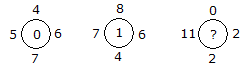# Verbal Reasoning - Character Puzzles - Discussion

### Discussion :: Character Puzzles - Character Puzzles 1 (Q.No.16)

16.

Which one will replace the question mark ?[A]. 0 [B]. 2 [C]. 11 [D]. 12

Explanation:

(6 + 5) - (7 + 4) = 0

and (7 + 6) - (8 + 4) = 1

Therefore (11 + 2) - (2 + 0) = 11.

 Padmaja said: (Dec 23, 2013) (7-6)-(5-4) = 0. (7-4)-(8-6) = 1. (11-0)-(2-2) = 11.

 Hitesify said: (Aug 30, 2016) (6 - 4) - (7 - 5) = 0, (6 - 8) - (4 - 7) = 1, (2 - 0) - (2 - 11) = 11.